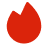# Oracle计算机科学面试真题

BQ

Coding（2）
System Design（0）
Algorithm（0）
Ood（0）高频题（0）

Coding（2）
System Design（0）
Algorithm（0）
Ood（0）高频题（0）
1.LeetCode 103
2.LeetCode 134
1. LeetCode 103
Given the root of a binary tree, return the zigzag level order traversal of its nodes' values. (i.e., from left to right, then right to left for the next level and alternate between).

Example 1:

```Input: root = [3,9,20,null,null,15,7]
Output: [,[20,9],[15,7]]
```
Example 2:

```Input: root = 
Output: []
```
Example 3:

```Input: root = []
Output: []
```

Constraints:

• The number of nodes in the tree is in the range [0, 2000].
• -100 <= Node.val <= 100
2. LeetCode 134
There are n gas stations along a circular route, where the amount of gas at the ith station is gas[i].

You have a car with an unlimited gas tank and it costs cost[i] of gas to travel from the ith station to its next (i + 1)th station. You begin the journey with an empty tank at one of the gas stations.

Given two integer arrays gas and cost, return the starting gas station's index if you can travel around the circuit once in the clockwise direction, otherwise return -1. If there exists a solution, it is guaranteed to be unique

Example 1:

```Input: gas = [1,2,3,4,5], cost = [3,4,5,1,2]
Output: 3
Explanation:
Start at station 3 (index 3) and fill up with 4 unit of gas. Your tank = 0 + 4 = 4
Travel to station 4. Your tank = 4 - 1 + 5 = 8
Travel to station 0. Your tank = 8 - 2 + 1 = 7
Travel to station 1. Your tank = 7 - 3 + 2 = 6
Travel to station 2. Your tank = 6 - 4 + 3 = 5
Travel to station 3. The cost is 5. Your gas is just enough to travel back to station 3.
Therefore, return 3 as the starting index.
```
Example 2:

```Input: gas = [2,3,4], cost = [3,4,3]
Output: -1
Explanation:
You can't start at station 0 or 1, as there is not enough gas to travel to the next station.
Let's start at station 2 and fill up with 4 unit of gas. Your tank = 0 + 4 = 4
Travel to station 0. Your tank = 4 - 3 + 2 = 3
Travel to station 1. Your tank = 3 - 3 + 3 = 3
You cannot travel back to station 2, as it requires 4 unit of gas but you only have 3.
Therefore, you can't travel around the circuit once no matter where you start.
```

Constraints:

• n == gas.length == cost.length
• 1 <= n <= 105
• 0 <= gas[i], cost[i] <= 104